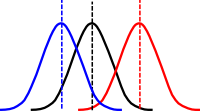# One-Way Analysis of Variance (ANOVA)

Analysis of variance is a statistical technique that is used to determine if there is a difference in two or more sample populations.  Z-test and t-tests areused when comparing one sample population to a known value or two sample populations to each other. When two or more sample populations are involved it is necessary to use analysis of variance.  The simple rule is 3 or more use analysis of variance

Analysis of variance is too complicated to do by hand, even though it is possible. It takes a great deal of time and one error will ruin the answer. Therefore, we are not going to look at equations during this example. Instead, we will focus on the hypotheses and practical applications of analysis of variance. To calculate analysis of variance results you can use SPSS or Microsoft excel.

There are several types of analysis of variance. We are going to first look at one-way analysis of variance.

Here are the assumptions for one-way analysis of variance

• Samples are randomly selected
• Samples are independently assigned
• Samples are homogeneous
• Sample is normally distributed

One-way analysis of variance is used when 2 or more groups receive the same treatment or intervention. The treatment is the independent variable while the means of each group is the dependent variable. This is because as the researcher, you control the treatment but you do not control the resulting mean that is recorded. One-way analysis of variance is often used in performing experiments.

Let’s look at an example. You want to know if there is any difference in the average life of four different breeds of dogs. You take a random sample of five dogs from four different breeds. Below are the results

Terrier    Retriever   Hound   Bulldog
12                 11                   12            12
13                 10                   11             15
14                 13                   15             10
11                 15                   15             12
15                 14                   16             11

In this example, the independent variable is the breed of dog. This is because you control this. You can select whatever dog breed you want. The dependent variable is the average length of the dog’s lives. You have no control over how long they live. You are trying to see if  dog breed influences how long the dog will live

Here are the hypotheses

Null hypotheses: There is no difference in the average length of a dog’s life because of breed

Alternative hypotheses: There is a difference in the average length of a dog’s life because of breed

The significance level is 0.05  are F critical is 3.24

After running the results in the computer we get an F-value of 0.76. This means we do not reject are null hypotheses.  This means that there is no difference in the average life of the dog breeds in this study.

One-way analysis is used when we have one treatment and three or more groups that experience the treatment. This statistical tool is useful for research designs that call on the need for experiments.